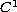Electron. J. Diff. Equ., Vol. 2009(2009), No. 113, pp. 1-26.

### Homeomorphisms and Fredholm theory for perturbations of nonlinear Fredholm maps of index zero with applications Petronije S. Milojevic

Abstract:
We develop a nonlinear Fredholm alternative theory involving k-ball and k-set perturbations of general homeomorphisms and of homeomorphisms that are nonlinear Fredholm maps of index zero. Various generalized first Fredholm theorems and finite solvability of general (odd) Fredholm maps of index zero are also studied. We apply these results to the unique and finite solvability of potential and semilinear problems with strongly nonlinear boundary conditions and to quasilinear elliptic equations. The basic tools used are the Nussbaum degree and the degree theories for nonlinear-Fredholm maps of index zero and their perturbations.

Submitted March 6, 2009. Published September 12, 2009.
Math Subject Classifications: 47H15, 35L70, 35L75, 35J40
Key Words: Nonlinear Fredholm mappings; index zero; homeomorphism; Fredholm alternative; potential problems; nonlinear boundary conditions; quasilinear elliptic equations.

Show me the PDF file (355 KB), TEX file, and other files for this article.Petronije S. Milojevic Department of Mathematical Sciences and CAMS New Jersey Institute of Technology Newark, NJ 07102, USA email: milojevi@adm.njit.edu